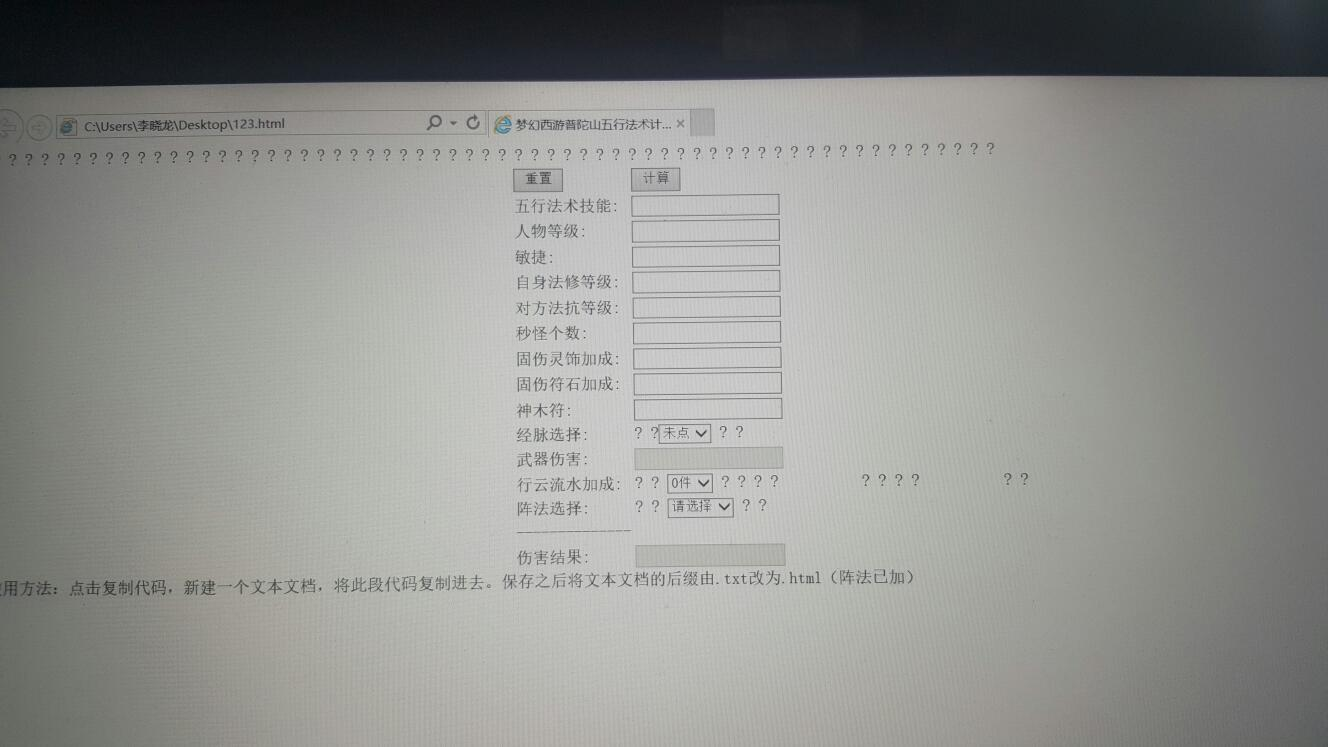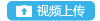登录 找回密码 注册通行证

【2017年4月门派调整】普陀五行学说对NPC伤害计算器[复制链接]发表于 2017-5-7 01:09:14 | 显示全部楼层 |阅读模式

马上注册，结交更多好友，享用更多功能，让你轻松玩转社区。

x

1. <!DOCTYPE html>
2. <html>
3. <meta name="content-type" content="text/html" >
5. <title>梦幻西游普陀山五行法术计算器</title>
6. <!--计算普陀山五行法术伤害-->
7. <script type="text/javascript">
8. var zhxg1=0;
9. var zhxg2=0;
10. var zhenfa=0;
11. var tuiyan=0;function check(obj){
12. if (isNaN(obj.value))
13. {
15.   obj.value="";
16. }
17. }
18. function selectzhenfa(){
19. var index1=document.getElementById("zf").selectedIndex;
20. if(index1 ==0)
21. {
22.   zhenfa = 0;
23. }
24. else if(index1 == 1)
25. {
26.   zhenfa = 20;
27. }
28. else if(index1 == 2)
29. {
30.   zhenfa = 15;
31. }
32. }
33. function selectjingmai(){
34. tuiyan=0;
35. var index1=document.getElementById("jingmai").selectedIndex;
36. if(index1==1)
37. {
38.   tuiyan=0;
39.   document.getElementById("wqsh").disabled=false;
40. }
41. else if(index1==2)
42. {
43.   tuiyan=50;
44.   document.getElementById("wqsh").disabled=true;
45. }
46. else
47. {
48.   tuiyan=0;
49.   document.getElementById("wqsh").disabled=true;
50. }
51. document.getElementById("wqsh").value = "";
52. }
53. function selectzh(){
54. zhxg1=0;
55. zhxg2=0;document.getElementById("zh1").options.selected = true;
56. document.getElementById("zh2").options.selected = true;
57. var index1=document.getElementById("zh").selectedIndex;
58. if(index1 == 0)
59. {
60.   document.getElementById("zh1").style.visibility="hidden";
61.   document.getElementById("zh2").style.visibility="hidden";
62. }
63. else if(index1 == 1)
64. {
65.   document.getElementById("zh1").style.visibility="visible";
66.   document.getElementById("zh2").style.visibility="hidden";
67. }
68. else if(index1 == 2)
69. {
70.   document.getElementById("zh1").style.visibility="visible";
71.   document.getElementById("zh2").style.visibility="visible";
72. }
73. }
74. function selectzh1(){
75. var index1=document.getElementById("zh1").selectedIndex;
76. if(index1 ==0)
77. {
78.   zhxg1 = 0;
79. }
80. else if(index1 == 1)
81. {
82.     zhxg1 = 25;
83. }
84. else if(index1 == 2)
85. {
86.     zhxg1 = 33;
87. }
88. else if(index1 == 3)
89. {
90.     zhxg1 = 50;
91. }
92. }
93. function selectzh2(){
94. var index1=document.getElementById("zh2").selectedIndex;
95. if(index1 ==0)
96. {
97.   zhxg2 = 0;
98. }
99. else if(index1 == 1)
100. {
101.     zhxg2 = 25;
102. }
103. else if(index1 == 2)
104. {
105.     zhxg2 = 33;
106. }
107. else if(index1 == 3)
108. {
109.     zhxg2 = 50;
110. }
111. }
112. function cz(){
113. zhxg1=0;
114. zhxg2=0;
115. zhenfa=0;
116. tuiyan=0;
117. document.getElementById("zh1").style.visibility="hidden";
118. document.getElementById("zh2").style.visibility="hidden";
119. document.getElementById("wqsh").disabled= true;
120. document.getElementById("jineng").value ="" ;
121. document.getElementById("minjie").value ="";
122. document.getElementById("dengji").value ="";
123. document.getElementById("lingshi").value ="";
124. document.getElementById("shenmuf").value ="";
125. document.getElementById("wqsh").value ="";
126. document.getElementById("geshu").value ="";
127. document.getElementById("faxiu").value ="";
128. document.getElementById("fakang").value ="";
129. document.getElementById("fushi").value ="";
130. document.getElementById("zsh").value ="";
131. document.getElementById("jingmai").options.selected = true;
132. document.getElementById("zh").options.selected = true;
133. document.getElementById("zh1").options.selected = true;
134. document.getElementById("zh2").options.selected = true;
135. document.getElementById("zf").options.selected = true;
136. }
137. function jssh(){
138. var jn ;
139. var mj ;
140. var dj ;
141. var ls ;
142. var smf;
143. var ws ;
144. var gs ;
145. var fx ;
146. var fk ;
147. var fs ;
148. var zsh;

149. if(document.getElementById("jineng").value =="") jn=0;
150. else jn =parseInt(document.getElementById("jineng").value);

151. if(document.getElementById("minjie").value =="") mj=0;
152. else mj =parseInt(document.getElementById("minjie").value);

153. if (document.getElementById("dengji").value =="") dj=0;
154. else dj =parseInt(document.getElementById("dengji").value);
155.
156. if (document.getElementById("lingshi").value =="") ls=0;
157. else ls =parseInt(document.getElementById("lingshi").value);

158. if (document.getElementById("shenmuf").value =="") smf=0;
159. else smf=parseInt(document.getElementById("shenmuf").value);

160. if (document.getElementById("wqsh").value =="") ws=0;
161. else ws =parseInt(document.getElementById("wqsh").value);
162. if (document.getElementById("geshu").value =="") gs=0;
163. else gs =parseInt(document.getElementById("geshu").value);
164. if (document.getElementById("faxiu").value =="") fx=0;
165. else fx =parseInt(document.getElementById("faxiu").value);
166. if (document.getElementById("fakang").value =="") fk=0;
167. else fk =parseInt(document.getElementById("fakang").value);
168. if (document.getElementById("fushi").value =="") fs=0;
169. else fs =parseInt(document.getElementById("fushi").value);
170. var zsh;
171. var xs;
172. if(gs>6||gs<=0)
173. {
175.   document.getElementById("geshu").value =="";
176. }
177. else
178. {
179.   if(gs==6)
180.   {
181.    xs=55
182.   }
183.   else
184.   {
185.    xs=(11-gs)*10
186.   }
187.
188.   zsh= parseInt(parseInt(parseInt((jn*3+50+parseInt((mj*7)/10)+parseInt(dj*zhxg1/100)+parseInt(dj*zhxg2/100)+ls+parseInt(ws*24/100))*xs/100)*(1+zhenfa/100))*(1+(fx-fk)*2/100))+(fx-fk)*5+parseInt(fs*(1+(fx-fk)*2/100))+parseInt(tuiyan)+parseInt(smf);
189.     document.getElementById("zsh").value=zsh;
190. }
191. }
192. </script>
194. <body>
195. <table align="center">
196.   <tr>
197.    <td><input type="submit" value="重置" onclick="cz()"/></td>
198.    <td><input type="submit" value="计算" onclick="jssh()"/></td>
199.   </tr>
200.   <tr>
201.    <td>五行法术技能:</td>
202.    <td><input type="text" id="jineng" onkeyup="check(this);"/></td>
203.   </tr>
204.   <tr>
205.    <td>人物等级:</td>
206.    <td><input type="text" id="dengji" onkeyup="check(this);"/></td>
207.   </tr>
208.   <tr>
209.    <td>敏捷:</td>
210.    <td><input type="text" id="minjie" onkeyup="check(this);"/></td>
211.   </tr>
212.   <tr>
213.    <td>自身法修等级:</td>
214.    <td><input type="text" id="faxiu" onkeyup="check(this);"/></td>
215.   </tr>
216.
217.   <tr>
218.    <td>对方法抗等级:</td>
219.    <td><input type="text" id="fakang" onkeyup="check(this);"/></td>
220.   </tr>
221.
222.   <tr>
223.    <td>秒怪个数:</td>
224.    <td><input type="text" id="geshu" onkeyup="check(this);"/></td>
225.   </tr>
226.
227.   <tr>
228.    <td>固伤灵饰加成:</td>
229.    <td><input type="text" id="lingshi" onkeyup="check(this);"/></td>
230.   </tr>
231.
232.   <tr>
233.    <td>固伤符石加成:</td>
234.    <td><input type="text" id="fushi" onkeyup="check(this);"/></td>
235.   </tr>
236.
237.   <tr>
238.    <td>神木符:</td>
239.    <td><input type="text" id="shenmuf" onkeyup="check(this);"/></td>
240.   </tr>
241.   <tr>
242.    <td>经脉选择:</td>
243.    <td>
244.    <select id="jingmai" onchange="selectjingmai()">
245.      <option value="0">未点</option>
246.      <option value="1">借灵</option>
247.      <option value="2">推衍</option>
248.    </select>
249.
250.    </td>
251.   </tr>
252.
253.   <tr>
254.    <td>武器伤害:</td>
255.    <td><input type="text" id="wqsh" onkeyup="check(this);" disabled="disabled"/></td>
256.   </tr>
257.
258.   <tr>
259.    <td>行云流水加成:</td>
260.    <td>
261.     <select id="zh" onchange="selectzh()">
262.      <option value="0">0件</option>
263.      <option value="1">1件</option>
264.      <option value="2">2件</option>
265.     </select>
266.
267.     <select id="zh1" onchange="selectzh1()" style="visibility:hidden">
268.      <option value="0">请选择</option>
269.      <option value="1">2孔</option>
270.      <option value="2">3孔</option>
271.      <option value="3">4孔</option>
272.     </select>
273.
274.     <select id="zh2" onchange="selectzh2()" style="visibility:hidden">
275.      <option value="0">请选择</option>
276.      <option value="1">2孔</option>
277.      <option value="2">3孔</option>
278.      <option value="3">4孔</option>
279.     </select>
280.    </td>
281.   </tr>
282.   <tr>
283.    <td>阵法选择:</td>
284.    <td>
285.     <select id="zf" onchange="selectzhenfa()">
286.      <option value="0">请选择</option>
287.      <option value="1">雷阵</option>
288.      <option value="2">天阵</option>
289.     </select>
290.    </td>
291.   </tr>
292.   <tr>
293.    <td>--------------</td>
294.   </tr>
295.   <tr>
296.    <td>伤害结果:</td>
297.    <td><input type="text" id="zsh" disabled="disabled"/></td>
298.   </tr>
299. </table>
300. </body>
301. </html>

评分迷糊の星星 + 20 感激不尽！虫虫° + 20 亲爱的,我才看到这个#24楼主| 发表于 2018-11-26 23:44:14| 显示全部楼层
 叼半根烟、想你 发表于 2018-11-26 12:43 乱码问题 楼主在标签里面加上就OK了 我想问一下，怎么解决计算出现小数就开始乱掉的问题啊，我平时写c的，取整很简单。用这个写，乘除出现小数之后就很乱，比如明明计算出来应该是整数的6，但是它就是会莫名其妙变成5.99999999999999导致最后有差异楼主| 发表于 2017-5-7 01:22:16| 显示全部楼层
 本帖最后由 °深冬蝶魂ミ 于 2017-5-8 13:02 编辑 网页版计算器传送门

评分发表于 2017-5-7 02:36:47| 显示全部楼层
 °深冬蝶魂ミ 发表于 2017-5-7 01:22 忘记加阵法效果了，算了明天再加 真是辛苦了！这么晚还不睡觉，熬夜易老哦评分

 [发帖际遇]: 倚窗赏飘雪 抢雷黑子的包子，被旺财重伤，看病花了 4 人气. 幸运榜 / 衰神榜梦幻玩家  发表于 2017-5-7 07:24:44厉害了楼主| 发表于 2017-5-7 09:57:45 | 显示全部楼层
 倚窗赏飘雪 发表于 2017-5-7 02:36 真是辛苦了！这么晚还不睡觉，熬夜易老哦帮忙看看计算结果对得上不
 [发帖际遇]: °深冬蝶魂ミ和黑山老妖在论坛上因发帖而相识，继而相知相恋，被他吸食了8 人气. 幸运榜 / 衰神榜发表于 2017-5-7 10:18:53 | 显示全部楼层
 为什么我点不出来计算结果

评分

 [发帖际遇]: 墨墨菡情在梦幻论坛拥有无数粉丝，这不，刚发帖又提升了 5 人气. 幸运榜 / 衰神榜楼主| 发表于 2017-5-7 10:20:30 | 显示全部楼层
 墨墨菡情 发表于 2017-5-7 10:18 为什么我点不出来计算结果 用IE打开。会提示是否允许啥啥脚本运行，要点允许
 [发帖际遇]: °深冬蝶魂ミ 在论坛发帖时没有注意，被紫衣盗偷去了 2 人气. 幸运榜 / 衰神榜楼主| 发表于 2017-5-7 10:21:21 | 显示全部楼层
 墨墨菡情 发表于 2017-5-7 10:18 为什么我点不出来计算结果 论坛之前好像抽风。我贴的代码里给我加了好多楼主| 发表于 2017-5-7 10:22:35 | 显示全部楼层
 墨墨菡情 发表于 2017-5-7 10:18 为什么我点不出来计算结果 这是我算的我自己的，对得上发表于 2017-5-7 12:14:41 | 显示全部楼层
 楼主好厉害，教我写JS吧评分楼主| 发表于 2017-5-7 13:31:46| 显示全部楼层
 道德无量天尊 发表于 2017-5-7 12:14 楼主好厉害，教我写JS吧 我是写c的，js我也不会写，这是百度出来慢慢凑的发表于 2017-5-7 13:37:43| 显示全部楼层
 为什么我ie打开点了允许还是不对

评分楼主| 发表于 2017-5-7 13:41:28| 显示全部楼层
 一只小莎莎 发表于 2017-5-7 13:37 为什么我ie打开点了允许还是不对 是界面都出不来，还是点计算没反应？发表于 2017-5-7 13:45:36| 显示全部楼层评分发表于 2017-5-7 13:46:17| 显示全部楼层
 °深冬蝶魂ミ 发表于 2017-5-7 13:41 是界面都出不来，还是点计算没反应？ 界面出来了，好多问好，计算不了结果

评分

 您需要登录后才可以回帖 登录 | 注册 本版积分规则 回帖后跳转到最后一页•••